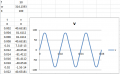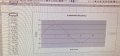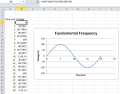# Triangular wave forms

#### John54321

Joined Apr 1, 2015
39
I have this final question on an assignment and i'm struggling, as this is self study course i need some help please.

Q. Synthesise and plot a triangular waveform up to and including the 7th harmonic using: v = 100 Sin (wt) as the fundamental for a frequency of 50Hz.

#### mxg2579

Joined Jun 10, 2015
15
Which part are you struggling with? Is it the 7th harmonic part that you have trouble with?

From your studies, you should recall that a harmonic is basically a multiple of the fundamental frequency of a signal. So the 2nd harmonic is:
v= 100 sin(2f*t), where f is the fundamental frequency. Since you have the frequency as 50 Hz (given), you should be able to plot these.

Does this help?

#### mxg2579

Joined Jun 10, 2015
15
Or is it the synthesize the triangle wave part you are confused with? Try and recall how a triangle wave can be created and you should have a better understanding.

#### MrAl

Joined Jun 17, 2014
6,460
Hi,

It sounds like you have to synthesize it from the basic harmonics, so you'll have to figure out the amplitudes and which harmonics stay and which dont, up to the 7th. If you have too much trouble i'll give you some hints. It's really easy if you just do a symmetrical triangle wave.

#### Veracohr

Joined Jan 3, 2011
688
Search for Fourier series of a triangle wave.

#### John54321

Joined Apr 1, 2015
39
Hi

Yes its the sums and how to get the points for the x-y scatter graph. Then use excel to plot the points as im not to familiar with excel formulas

Thanks

#### Ramussons

Joined May 3, 2013
797
As Veracohr said, If you study the Fourier Analysis of a Triangular Wave, you will know about the Harmonics Present and their Amplitudes.

Fourier Analysis is Splitting the original wave into its harmonics. Do the Reverse#### Veracohr

Joined Jan 3, 2011
688
Hi

Yes its the sums and how to get the points for the x-y scatter graph. Then use excel to plot the points as im not to familiar with excel formulas

Thanks
This is what I do: make a column for time which increments by some appropriate amount based on the frequency you choose, and extends for whatever length of time/number of cycles you want to plot. Make a column for the formula which you type in. You already have the only function you need to use in the formula; type it in the top cell and fill down to the bottom. Just make sure you use parentheses to group terms, and use * as the multiplication function. π in Excel has to be typed as pi().

I like to put terms such as ω in a single cell and reference it; if you do this make sure you anchor the reference (ie. cell B2 in your formula should appear as $B$2. You can do this quickly if you're using Microsoft Excel by pressing F4 when the cursor is on the cell name in the formula; it may not work that way in other spreadsheet programs.) Then select the range from the top of the t column (including the 't') to the bottom of the v column and choose Insert->Scatter (I use smooth lines usually).•GopherT and John54321

#### John54321

Joined Apr 1, 2015
39
Hi Veracohr

I will try and sort the formulas and get the correct results.

Kind regards

John

#### John54321

Joined Apr 1, 2015
39
Hi Veracohr

I will try and sort the formulas and get the correct results.

Kind regards

John
This is what I do: make a column for time which increments by some appropriate amount based on the frequency you choose, and extends for whatever length of time/number of cycles you want to plot. Make a column for the formula which you type in. You already have the only function you need to use in the formula; type it in the top cell and fill down to the bottom. Just make sure you use parentheses to group terms, and use * as the multiplication function. π in Excel has to be typed as pi().

I like to put terms such as ω in a single cell and reference it; if you do this make sure you anchor the reference (ie. cell B2 in your formula should appear as $B$2. You can do this quickly if you're using Microsoft Excel by pressing F4 when the cursor is on the cell name in the formula; it may not work that way in other spreadsheet programs.) Then select the range from the top of the t column (including the 't') to the bottom of the v column and choose Insert->Scatter (I use smooth lines usually).

View attachment 87363

Hi Veracohr

I have the voltages at the differnet harmonics but am struggling to get them in excel the 3rd harmonic should be 11v the 5th harmonic 4v and the 7th harmonic will be 2v. How would you put this into excel as im no good at excel and drawing by hand would'nt look good

Regards

John

#### Veracohr

Joined Jan 3, 2011
688
Hi Veracohr

I have the voltages at the differnet harmonics but am struggling to get them in excel the 3rd harmonic should be 11v the 5th harmonic 4v and the 7th harmonic will be 2v. How would you put this into excel as im no good at excel and drawing by hand would'nt look good
v = 100 Sin (wt)
You have the function right there, at a magnitude of 100 for the fundamental. Just replace 100 with the other magnitudes for the harmonics.

How about you type out the full equation here as you understand it?

#### WBahn

Joined Mar 31, 2012
24,684
You want to have multiple columns in your spreadsheet, one for each harmonic, then add them all up horizontally.

So the first column would be time, the second column would be the first harmonic, the third column would be the second harmonic, and so on. Then the eighth column would be the seventh harmonic and the ninth column would be the sum of the second through eight columns.

To make life easy, control each column with cells containing the amplitude and frequency at the top of the column and use absolute/relative referencing for the formula in main computation cells. That way you only have to write one main formula and simply copy it down the column for each time step and then copy the column to the right for each harmonic.

#### John54321

Joined Apr 1, 2015
39
Hi Veracohr

These were the calculations on the attached pic

3rd harmonic = A3 = 1/3sqt 100 sin (wt)
5th harmonic = A5 = 1/5sqt 100 sin (wt)
7th harmonic = A7 = 1/7sqt 100 sin (wt)

Last edited:

#### Veracohr

Joined Jan 3, 2011
688
OK, so sum those three equations and you have your waveform. Like Wbahn said, you can put each equation into its own column, then add the three columns together and plot.

#### John54321

Joined Apr 1, 2015
39
I wish I knew how to use excel that's the struggle. I never use it.

#### WBahn

Joined Mar 31, 2012
24,684
I wish I knew how to use excel that's the struggle. I never use it.

There are plenty of online tutorials on how to use Excel. For what you are trying to do you only need some very basics.

#### John54321

Joined Apr 1, 2015
39
There are plenty of online tutorials on how to use Excel. For what you are trying to do you only need some very basics.

Thanks Wbahn I will have a look on u tube.

#### John54321

Joined Apr 1, 2015
39

#### WBahn

Joined Mar 31, 2012
24,684
Do you REALLY need a 2.5 MB file?

Instead of taking a picture of your computer display, how about just doing a screen capture of it.

Use the Print Screen key to copy an image of your screen to the clip board and then open Paint and paste it in. Then crop the image to the part you want and resize it to something reasonable. Doing all of that to the poor-quality image you posted took about two minutes and yielded:Which is less than 100 kB.

If you capture from Excel directly you get something like this for only 67 kB:As for whether it is correct, well, does it look like 100V·sin (wt) where the frequency is 50 Hz?

#### John54321

Joined Apr 1, 2015
39
Do you REALLY need a 2.5 MB file?

Instead of taking a picture of your computer display, how about just doing a screen capture of it.

Use the Print Screen key to copy an image of your screen to the clip board and then open Paint and paste it in. Then crop the image to the part you want and resize it to something reasonable. Doing all of that to the poor-quality image you posted took about two minutes and yielded:

View attachment 89014

Which is less than 100 kB.

If you capture from Excel directly you get something like this for only 67 kB:

View attachment 89017

As for whether it is correct, well, does it look like 100V·sin (wt) where the frequency is 50 Hz?
Hi Wbahn

I was having trouble doing what you said so did it using the phone. Yes it looks correct im just making sure before i work the other formulas out for the 3rd 5th and 7th harmonics. Like i said i never use Excel so its just piece of mind its ok.

Thanks